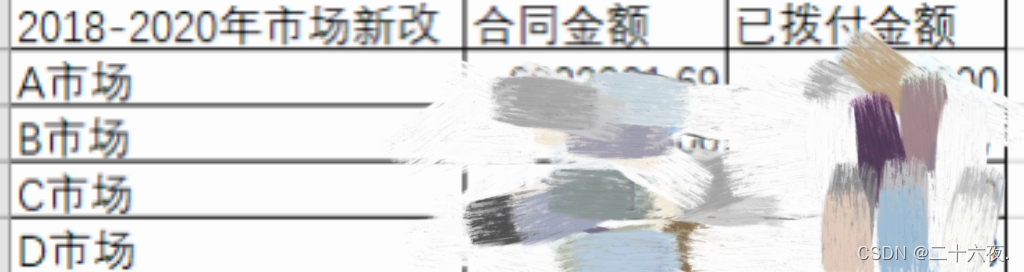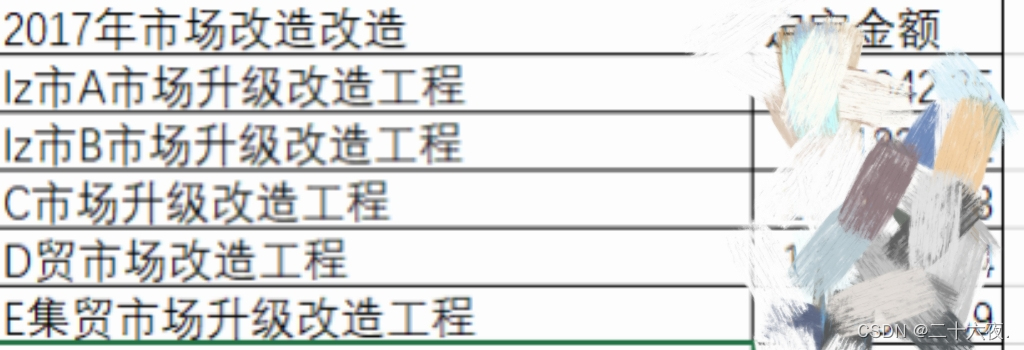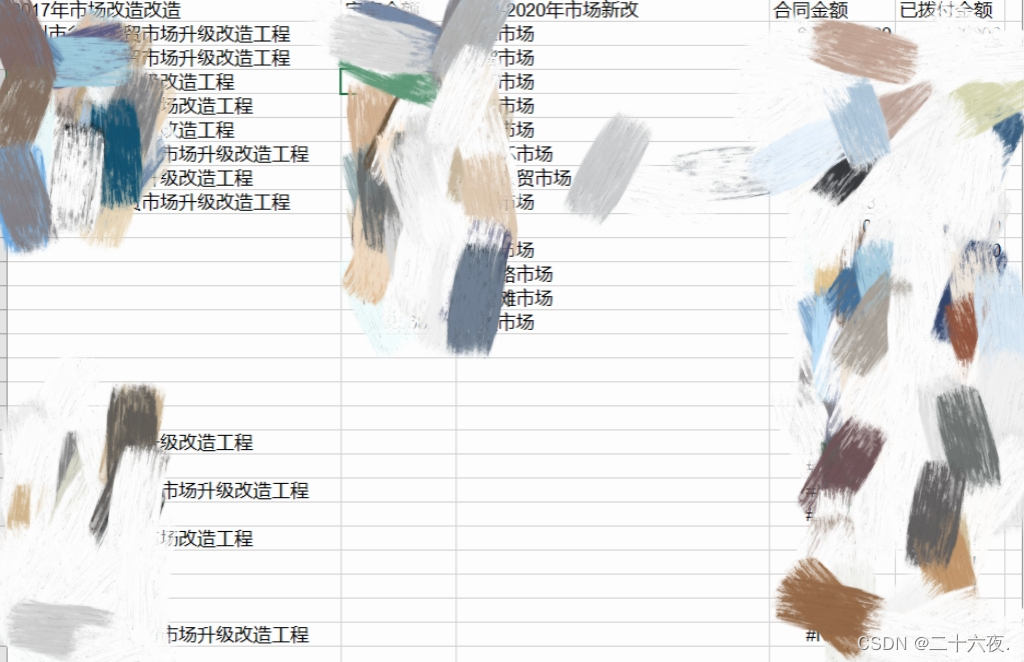3.1

3.2

3.3

3.4

3.5

# 三、代码编写

## 3.1

``````df1=pd.read_excel(r'D:\杂货\项目.xlsx',sheet_name='Sheet1')两个表格的内容形式大致如上。而我的需求是将这两个表格相关的工程项目匹配归类。

https://blog.csdn.net/Deng333333555/article/details/125092526?spm=1001.2014.3001.5501

## 3.2

``````for i in df1['XXXXXX改造']:#将这两列的数据存入list1和list2两个列表中
list1.append(i)
for j in df2['XXXXXX新改']:
list2.append(j)``````

## 3.3

``````for n in range(len(list2)):#通过模糊匹配算法，将list2与list1中的数据一一匹配，设置近似度为42%，得到匹配结果res
query_word=str(list2[n])
res=difflib.get_close_matches(query_word,list1,1,cutoff=0.42)
res = "".join(res)
listx.append(res)
``````

## 3.4

``````for m in range(len(list1)):#同上，将list1与list2的数据一一匹配
query_word=str(list1[m])
res=difflib.get_close_matches(query_word,list2,1,cutoff=0.42)
res="".join(res)
listy.append(res)``````

## 3.5

``````workbook=xlwt.Workbook(encoding='utf-8')#设定好新的excel表格的参数
worksheet.write(0,0,label='XXX改造')#从第0行第0列开始输入标签为XXX改造的数据
worksheet.write(0,1,label='XX金额')#从第0行第1列开始输入标签为XX金额的数据
worksheet.write(0,2,label='XXX新改')
worksheet.write(0,3,label='XX金额')
worksheet.write(0,4,label='已XXX金额')

for i in range(len(listx)):#写入运算出来的数据
worksheet.write(i+1,0,label=listx[i])
for j in range(len(listy)):
worksheet.write(j+1,2,label=listy[j])
for k in range(len(list1)):
worksheet.write(k+1,1,label=list3[k])
for l in range(len(list2)):
worksheet.write(l+1,3,label=list4[l])
worksheet.write(l+1,4,label=list5[l])
workbook.save(r'D:\杂货\新项目6.xls')#导出excel表格
``````通过两遍匹配，两者相互匹配度都高的则会出对应地出现在表格中，而只有单一匹配度高的，则出现了左边有数据右边没有数据，或者右边有数据左边没有数据的情况。

# 四、代码集合

``````import pandas as pd
import difflib
import xlwt#导入库

list1=[]#设置空列表，用于存储2017年一列的数据
list2=[]#用于存储2018年一列的数据
list3=list(df1['XX金额'])#将excel表格中的列数据列表化
list4=list(df2['XX金额'])
list5=list(df2['XXX金额'])
listx=[]#用于存储匹配结果的数据
listy=[]#同上
for i in df1['XXXXXXXXX改造']:#将这两列的数据存入list1和list2两个列表中
list1.append(i)
for j in df2['XXXXXXXXXXXXX新改']:
list2.append(j)

for n in range(len(list2)):#通过模糊匹配算法，将list2与list1中的数据一一匹配，设置近似度为42%，得到匹配结果res
query_word=str(list2[n])
res=difflib.get_close_matches(query_word,list1,1,cutoff=0.42)
res = "".join(res)
listx.append(res)

for m in range(len(list1)):#同上，将list1与list2的数据一一匹配
query_word=str(list1[m])
res=difflib.get_close_matches(query_word,list2,1,cutoff=0.42)
res="".join(res)
listy.append(res)

workbook=xlwt.Workbook(encoding='utf-8')#设定好新的excel表格的参数
worksheet.write(0,0,label='XXXXXXXXX改造')
worksheet.write(0,1,label='XX金额')
worksheet.write(0,2,label='XXXXXXXXXXX新改')
worksheet.write(0,3,label='XX金额')
worksheet.write(0,4,label='XXX金额')

for i in range(len(listx)):#写入运算出来的数据
worksheet.write(i+1,0,label=listx[i])
for j in range(len(listy)):
worksheet.write(j+1,2,label=listy[j])
for k in range(len(list1)):
worksheet.write(k+1,1,label=list3[k])
for l in range(len(list2)):
worksheet.write(l+1,3,label=list4[l])
worksheet.write(l+1,4,label=list5[l])
workbook.save(r'D:\杂货\新项目6.xls')#导出excel表格
``````

# 五、总结